# 数据结构与算法之美学习笔记

## 基础篇之排序(上)

Posted by Alee on October 14, 2019

## 基础篇之排序(上)

#### 如何分析一个“排序算法”

• 排序算法的执行效率

对于排序算法执行效率的分析，从这几个方面来衡量：

1. 最好情况、最坏情况、平均情况时间复杂度

在分析排序算法的时间复杂度时，要分别给出最好情况、最坏情况、平均情况下的时间复杂度，还得说出最好、最坏时间复杂度对应的要排序的原始数据是什么样的。

为什么要区分这三种时间复杂度呢？第一，有些排序算法会区分，为了好对比，我们最好都做一下区分。第二，对于要排序的数据，有的接近有序，有的完全无序。有序度不同的数据，对于排序的执行时间肯定是有影响的，我们要知道排序算法在不同数据下的性能表现。

2. 时间复杂度的系数、常数、低阶

时间复杂度反映的是数据规模n很大的时候的一个增长趋势，所以它表示的时候会忽略系数、常数、低阶。但实际软件开发中，排序的可能是10个、100个、1000个这样规模很小的数据，所以，在对同一阶时间复杂度的排序算法性能比较的时候，我们就要把系数、常数、低阶也考虑进来。

3. 比较次数和交换（移动）次数

基于比较的排序算法的执行过程，会涉及两种操作，一种是元素比较大小，另一种是元素交换或移动。所以，在分析排序算法的执行效率的时候，应该把比较次数和交换次数也考虑进去。

• 排序算法的内存消耗

算法的内存消耗可以通过空间复杂度来衡量，排序算法也不例外。针对排序算法的空间复杂度，有一个新的概念，原地排序(Sorted in place)。原地排序算法，就是特指空间复杂度是O(1)的排序算法。

• 排序算法的稳定性

仅仅用执行效率和内存消耗来衡量排序算法的好坏是不够的。针对排序算法，有一个重要的度量指标，稳定性。这个概念是说，如果待排序的序列中存在值相等的元素，经过排序之后，相等元素之间原有的先后顺序不变

举个例子：比如我们有一组数据 2，9，3，4，8，3，按照大小排序之后就是 2，3，3，4，8，9。

这组数据里有两个3，经过某种排序算法排序之后，如果两个3的前后顺序没有改变，那么这种排序算法就叫作稳定的排序算法；如果前后顺序发生变化，那就叫作不稳定的排序算法

或许你有疑问，两个3哪个在前，哪个在后有什么关系呢？稳不稳定又有什么关系呢？为什么要考察排序算法的稳定性呢？

在真正软件开发中，要排序的往往不是单纯的整数，而是一组对象。需要按照对象的某个key来排序。

比如：现在要给电商交易系统中的“订单”排序。订单有两个属性，一个是下单时间，另一个是订单金额。如果现在有10万条订单数据，希望按照金额从小到大对订单数据排序。对于金额相同的订单，希望按照下单时间从早到晚排序。对于这样一个排序需求，该如何实现呢？

最先想到的是：先按照金额对订单数据进行排序，然后，再遍历排序之后的订单数据，对于每个金额相同的小区间再按照下单时间排序。这种排序思路理解起来简单，但实现起来会很复杂。

而借助稳定排序算法可以非常简洁地解决。解决思路是：先按照下单时间给订单排序，注意是按照下单时间，不是金额。排序完成之后。再用稳定排序算法，按照订单金额重新排序。两遍排序之后，得到的订单数据就是按照金额从小到大排序，金额相同的订单按照下单时间从早到晚排序的。为什么呢？

稳定排序算法可以保持金额相同的两个对象，在排序之后的前后顺序不变。第一次排序之后，所有的订单按照下单时间从早到晚有序了。在第二次排序中，我们用的是稳定的排序算法，所以经过第二次排序后，相同金额的订单仍然保持下单时间从早到晚有序。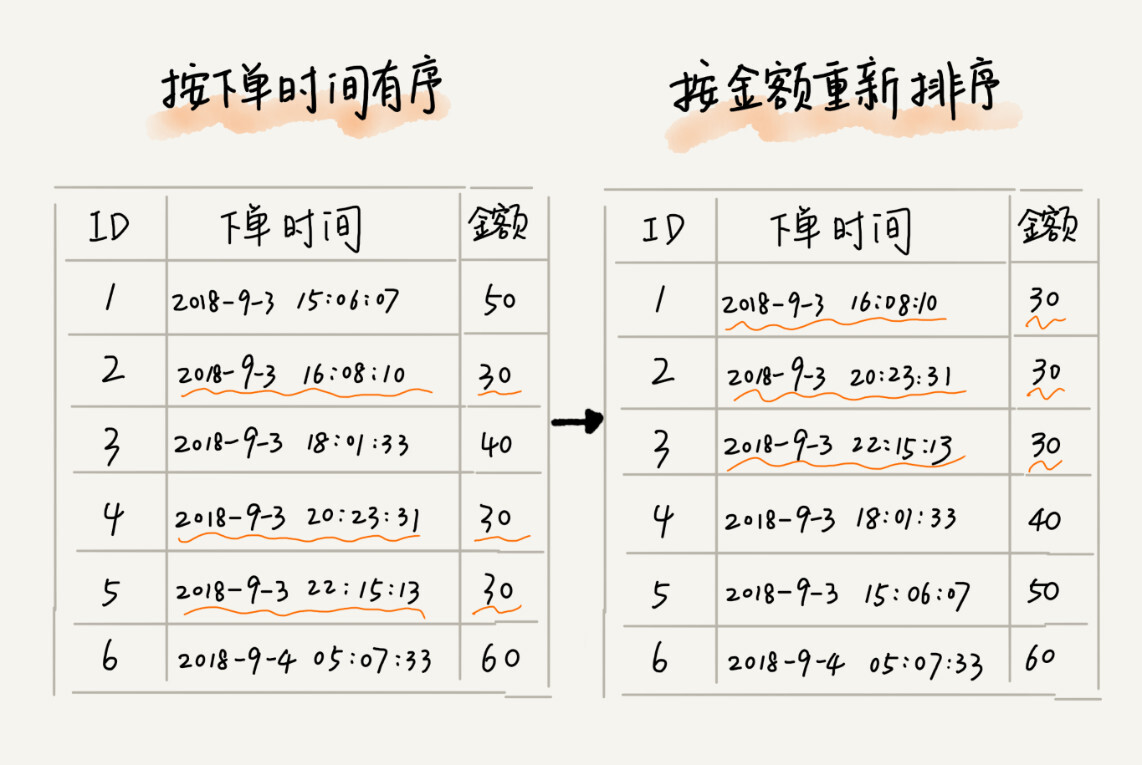#### 冒泡排序(Bubble Sort)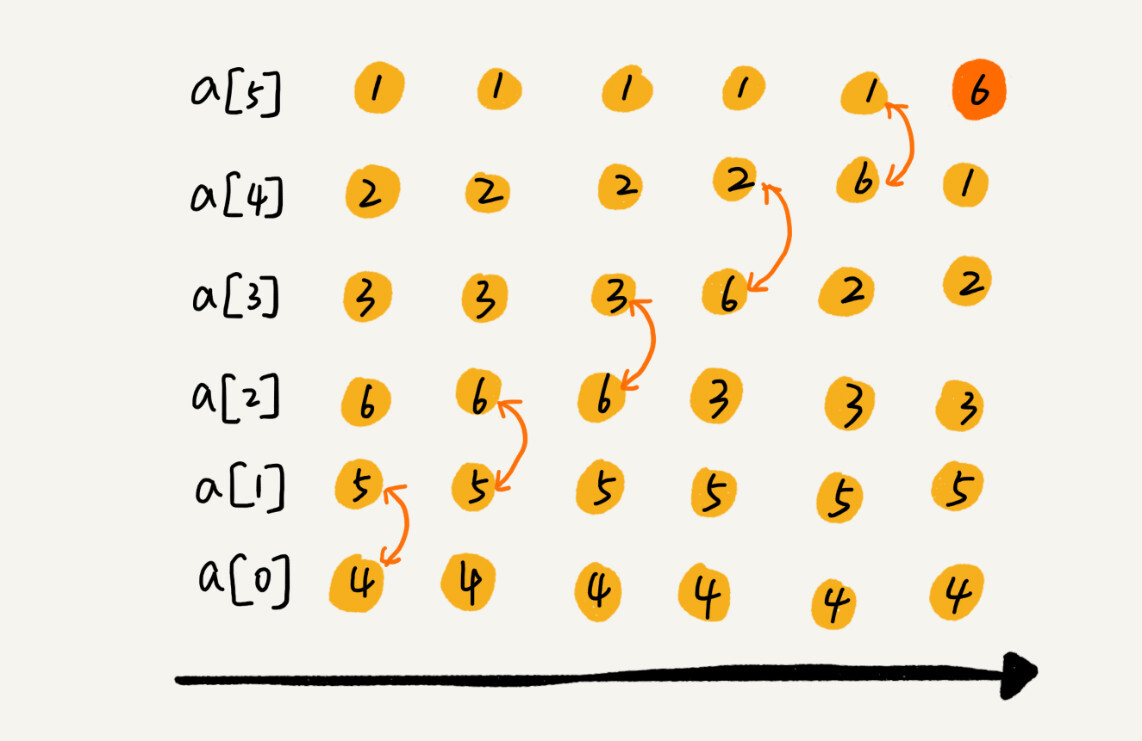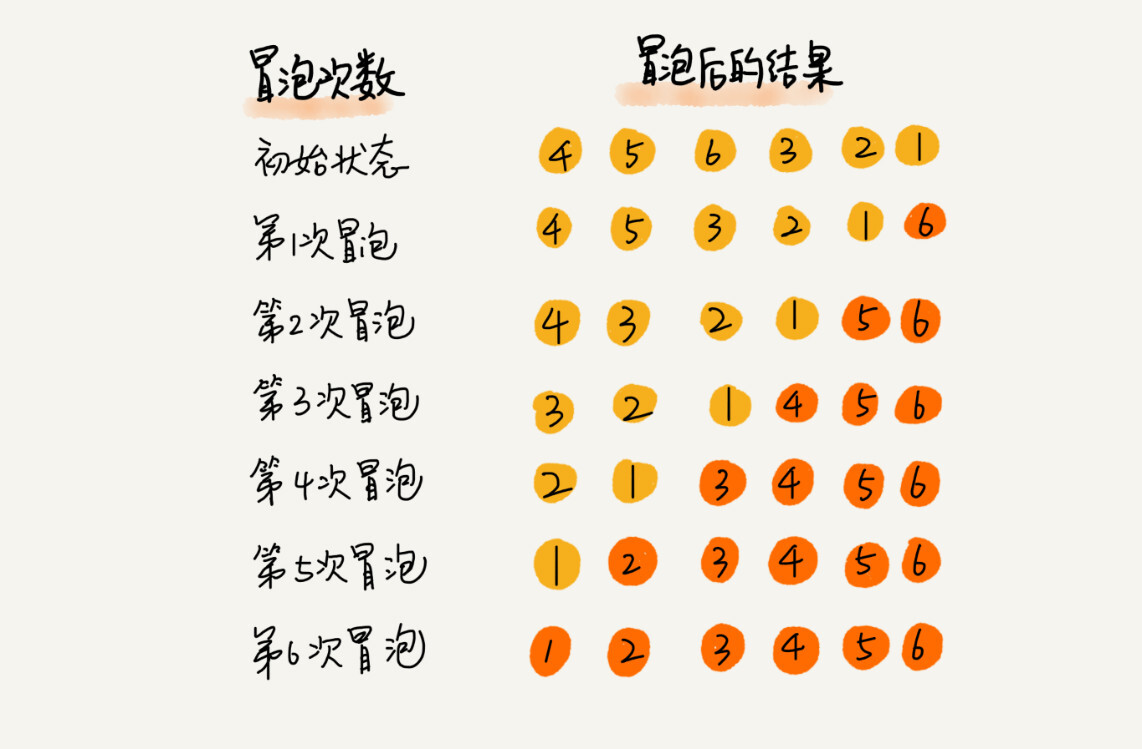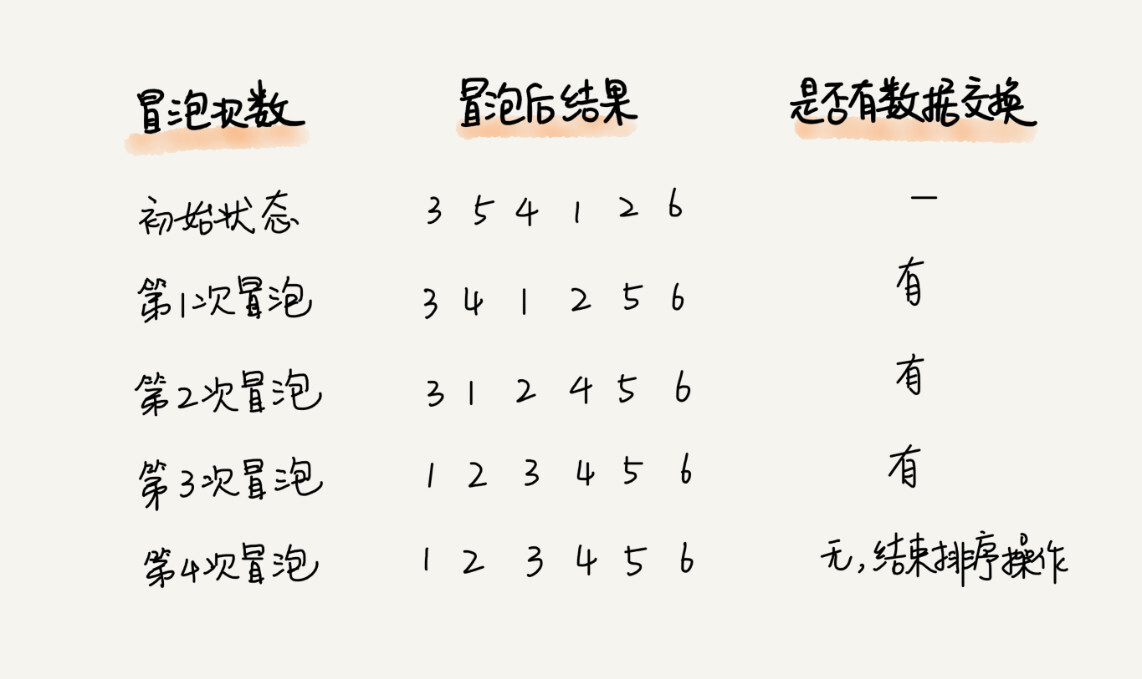``````//冒泡排序，a 表示数组， n 表示数组大小
public void bubbleSort(int[] a, int n) {
if (n <= 1) return;
for (int i = 0; i < n; i++) {
//提前退出冒泡循环的标志位
boolean flag = false;
for (int j = 0; j < n - i - 1; j++) {
if (a[j] > a[j+1]) {
int temp = a[j];
a[j] = a[j+1];
a[j+1] = temp;
flag = true; //表示有数据交换
}
}
if (!flag) break; //没有数据交换，提前退出。
}
}
``````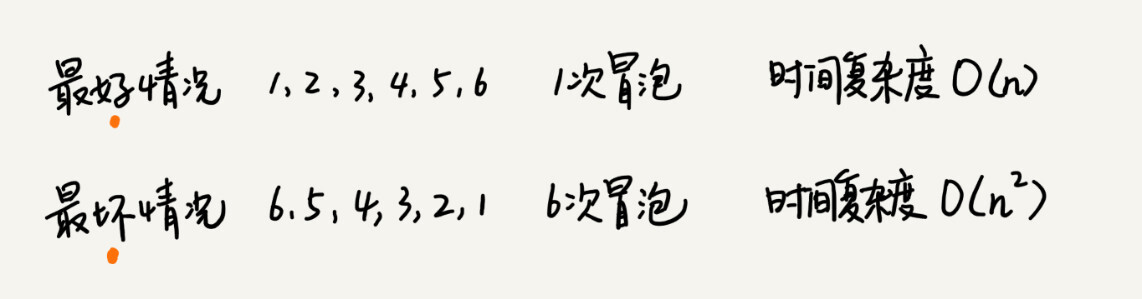``````有序元素对: a[i] <= a[j], 如果 i < j。
``````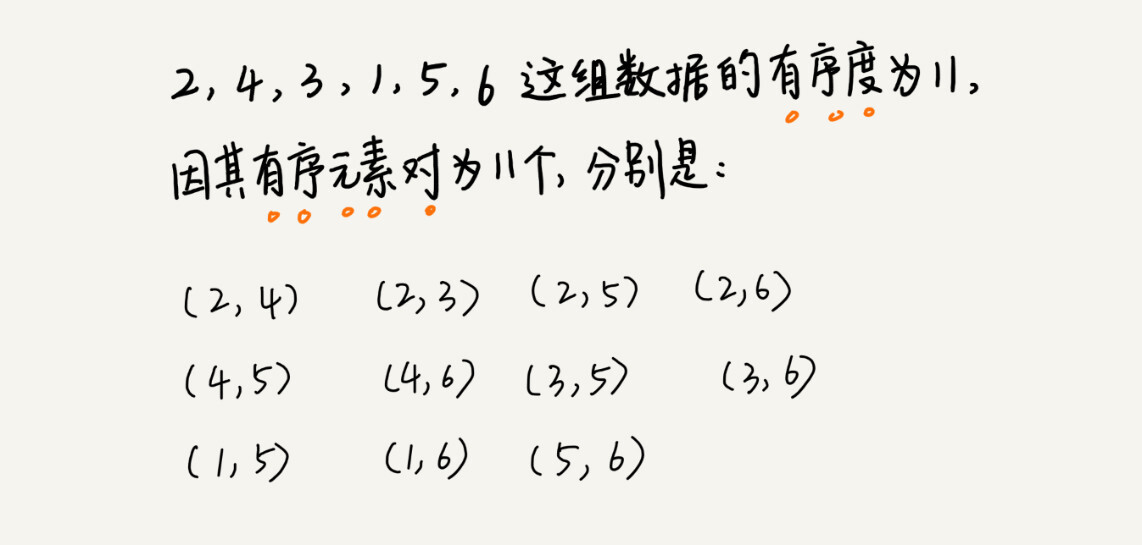``````逆序元素对: a[i] > a[j], 如果 i < j。
``````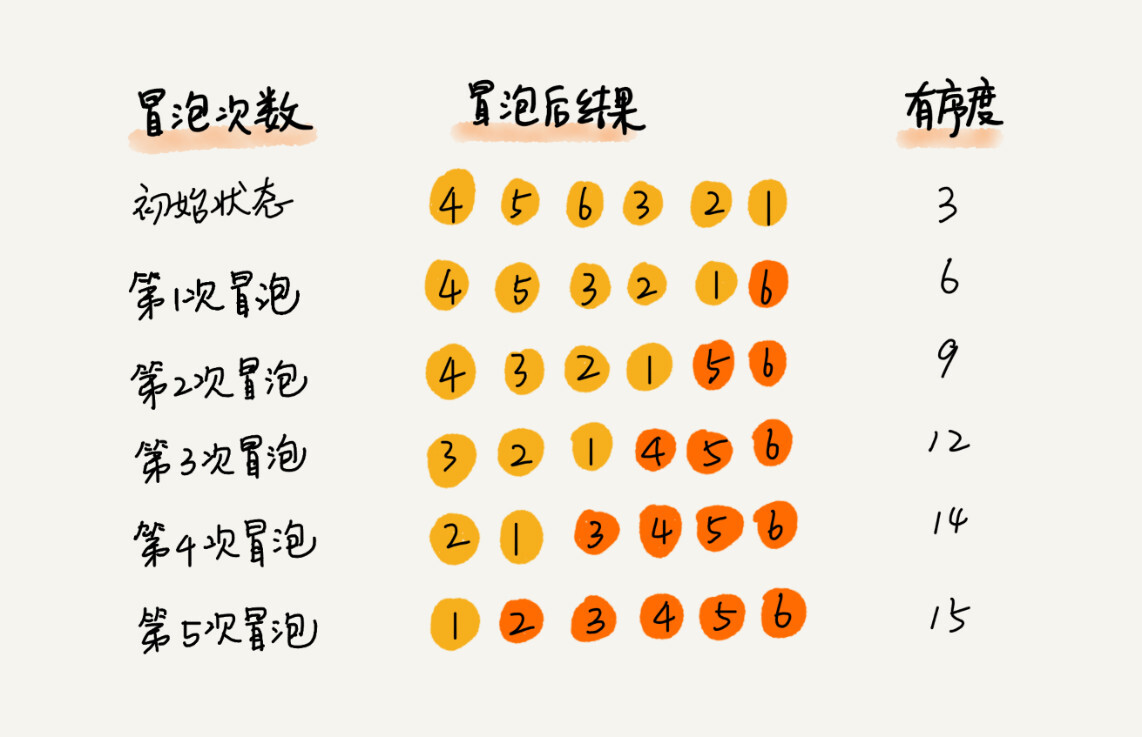#### 插入排序(Insertion Sort)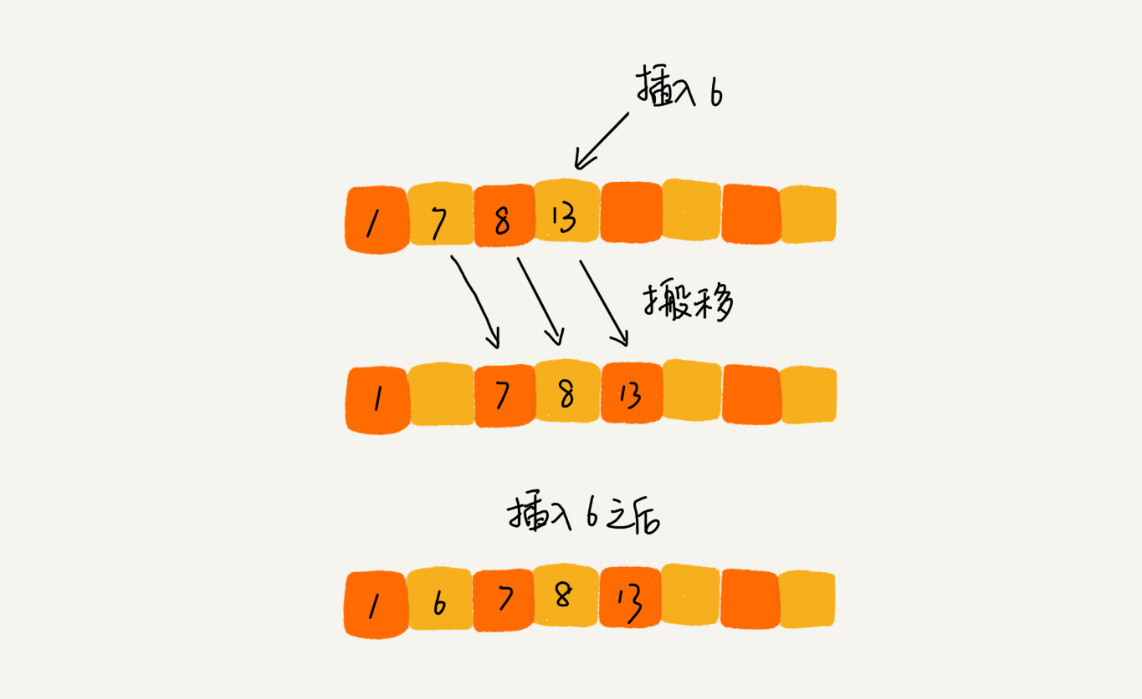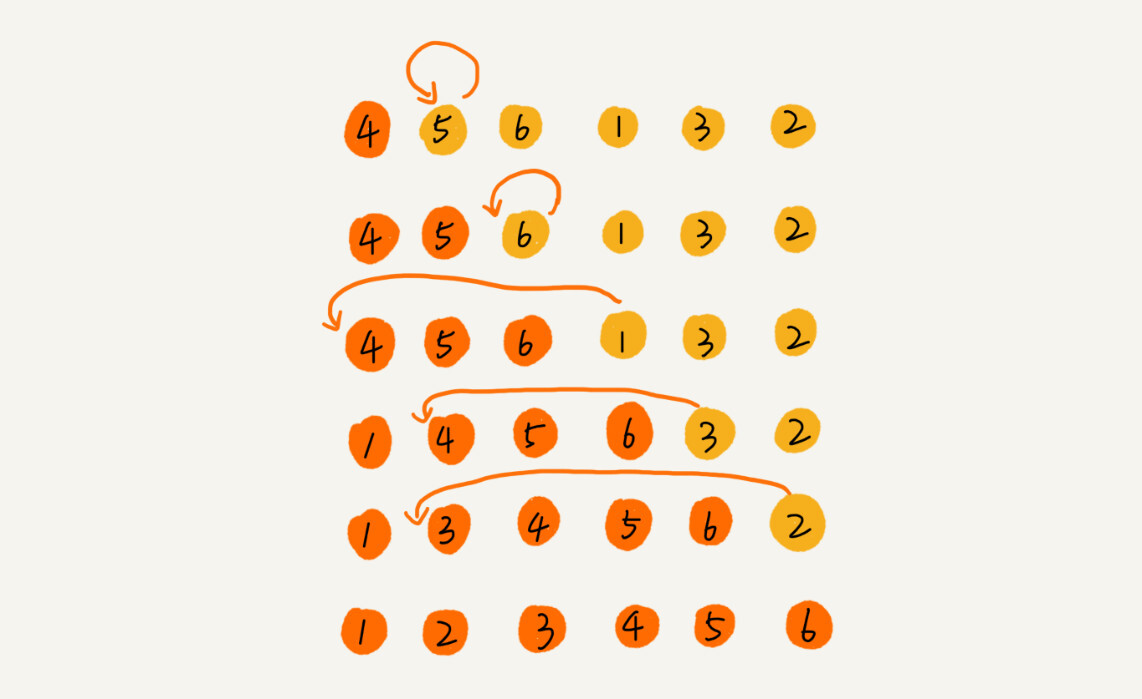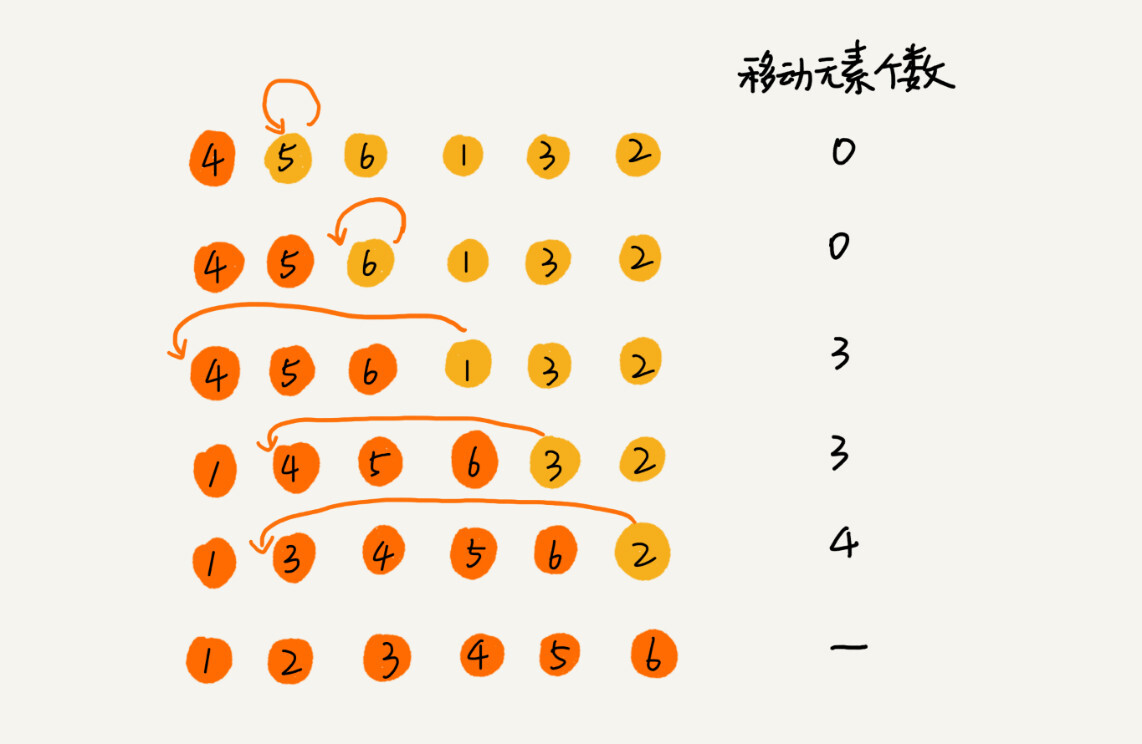``````//插入排序， a 表示数组， n 表示数组大小
public void insertionSort(int[] a, int n) {
if (n <= 1) return;

for (int i = 1; i < n; i++) {
int value = a[i];
int j = i - 1;
//查找插入的位置。
for (; j >= 0; j--) {
if (a[j] > value) {
a[j+1] = a[j]; //移动数据
} else {
break;
}
}
a[j+1] = value; //插入数据
}
}
``````

#### 选择排序(Selection Sort)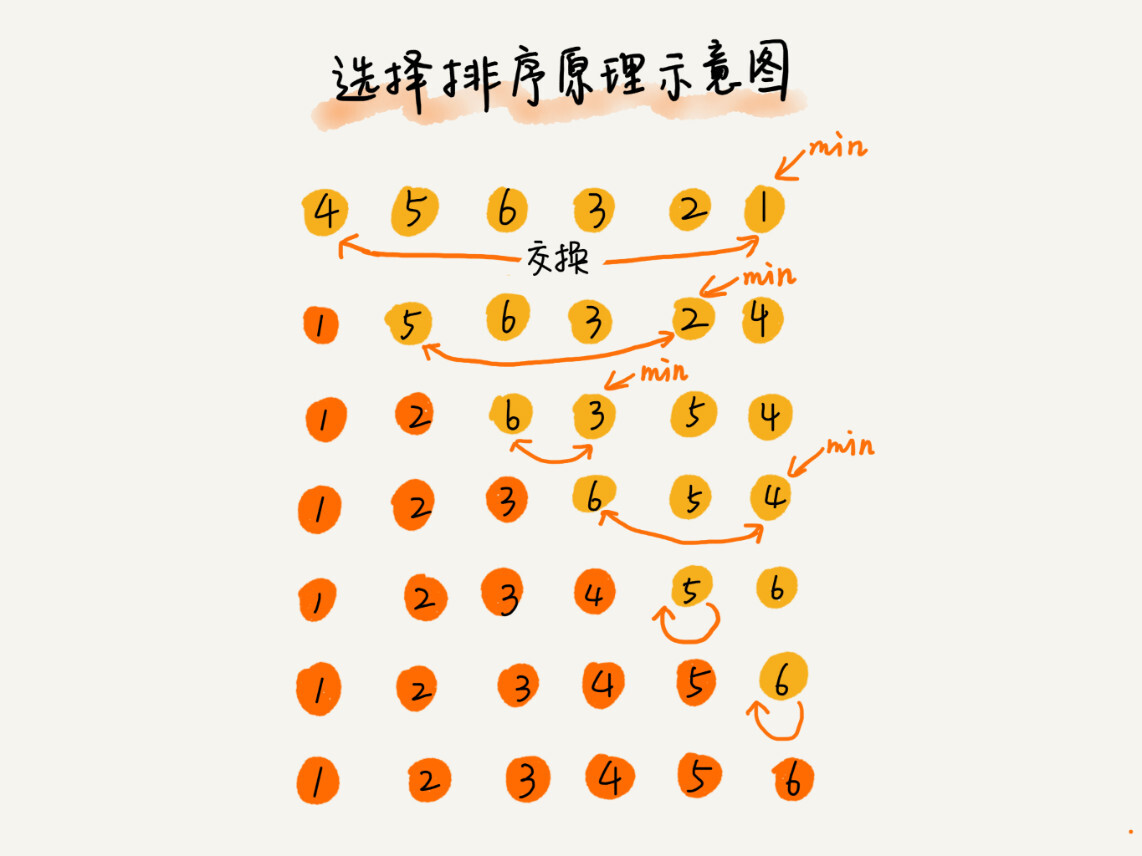``````//选择排序， a 表示数组， n 表示数组大小
public static void seletcionSort(int[] a, int n) {
if (n <= 1) return;

for (int i = 0; i < n; i++) {
int min = i;
for (int j = i + 1; j < n; j++) {
if (a[j] < a[min]) {
min = j;
}
}
//数据交换
if (min != i) {
int temp = a[min];
a[min] = a[i];
a[i] = temp;
}
}
}
``````

#### 解答开篇

``````//冒泡排序中数据的交换操作：
if (a[j] > a[j+1]) { // 交换
int tmp = a[j];
a[j] = a[j+1];
a[j+1] = tmp;
flag = true;
}

//插入排序中数据的移动操作：
if (a[j] > value) {
a[j+1] = a[j];  // 数据移动
} else {
break;
}
``````

``````import java.util.*;

class Alee {
public static void main(String[] args) {
Random random = new Random();
//随机生成10000个数组
for (int i = 0; i < 10000; i++) {
int[] temp = new int;
for (int j = 0; j < 200; j++) {
temp[j] = random.nextInt();
}
//使用冒泡排序 大约需要750ms
bubbleSort(temp, 200);
//使用插入排序 只需要280ms左右
//			insertionSort(temp, 200);
}
}

//冒泡排序
public static void bubbleSort(int[] a, int n) {
if (n <= 1) return;
for (int i = 0; i < n; i++) {
//提前退出冒泡循环的标志位
boolean flag = false;
for (int j = 0; j < n - i - 1; j++) {
if (a[j] > a[j+1]) {
int temp = a[j];
a[j] = a[j+1];
a[j+1] = temp;
flag = true; //表示有数据交换
}
}
if (!flag) break; //没有数据交换，提前退出。
}
}

//插入排序
public static void insertionSort(int[] a, int n) {
if (n <= 1) return;

for (int i = 1; i < n; i++) {
int value = a[i];
int j = i - 1;
//查找插入的位置。
for (; j >= 0; j--) {
if (a[j] > value) {
a[j+1] = a[j]; //移动数据
} else {
break;
}
}
a[j+1] = value; //插入数据
}
}
}
``````

``````//希尔排序
public static void shellSort(int[] arr) {
int length = arr.length;
int temp;
for (int step = length / 2; step >= 1; step /= 2) {
for (int i = step; i < length; i++) {
temp = arr[i];
int j = i - step;
while (j >= 0 && arr[j] < temp) {
arr[j + step] = arr[j];
j -= step;
}
arr[j + step] = temp;
}
}
}
``````

#### 内容小结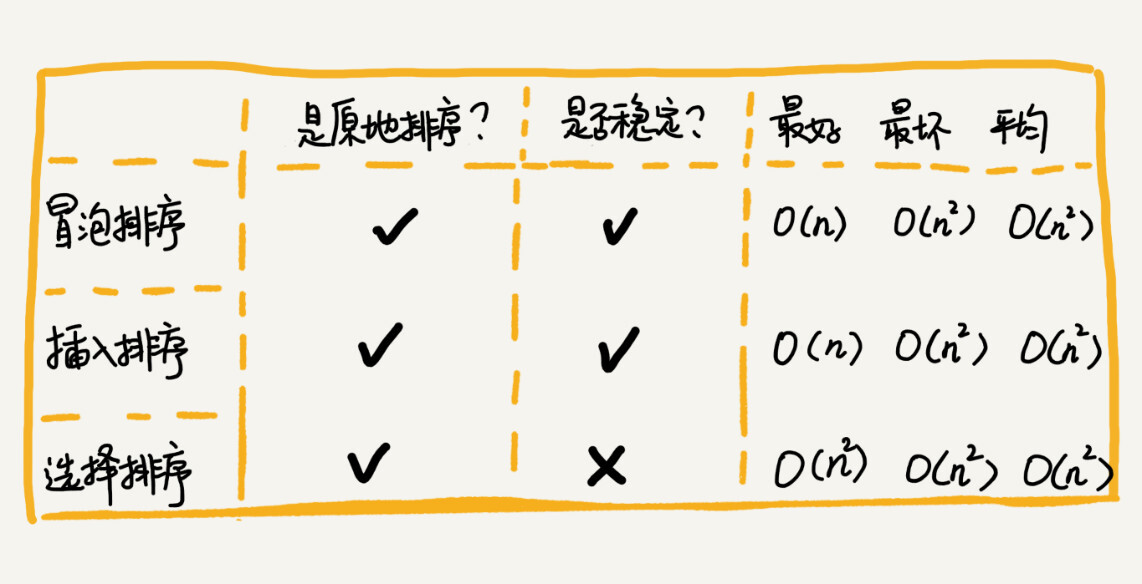#### 思考

1. 如果只考虑改变节点位置，不修改链表节点的value值，冒泡排序相比于数组实现，比较次数一致，但是交换操作更复杂；插入排序，比较次数一致，不需要再有后移操作，找到位置后可以直接插入，但排序完毕后可能需要倒置链表；选择排序比较次数一致，交换操作同样比较麻烦。所以，时间复杂度和空间复杂度并无明显变化，如果追求极致性能，冒泡排序的时间复杂度系数会变大，插入排序系数会减小，选择排序无明显变化。